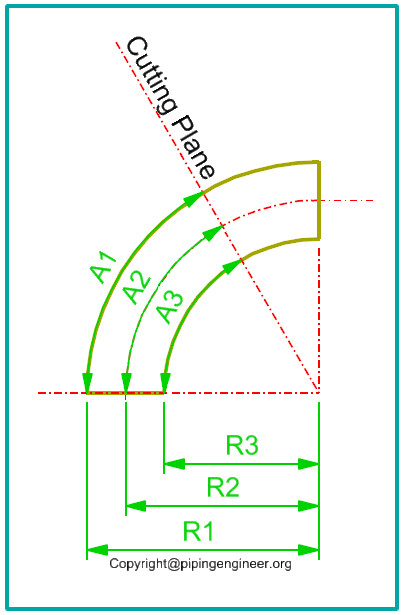# Custom Degree Elbow Cutting Calculator

Many times at site, custom degree elbows are required apart from regular 45 Degree and 90 Degree elbows. This is achieved by cutting available 45 degree or 90 degree elbow.This requires a circular marking on elbow for which three dimensions are necessary to be calculated.

1. A1 : Circular distance along inside surface of elbow from reference edge of elbow.
2. A2 : Circular distance along side of elbow at elbow center location, from reference edge of elbow.
3. A3: Circular distance along outside surface of elbow from reference edge of elbow.In above figure,

1. R1 : Outside surface radius of elbow, which is equal to elbow center radius + half of OD of elbow.
2. R2 : Elbow center radius, usually 1.5 times of nominal pipe diameter of elbow for long radius elbow or 3 times of nominal pipe diameter for 3D elbows.
3. R3 : Inside surface radius of elbow, which is equal to elbow center radius – half of OD of elbow.

We know that circumference of a circle is equal to Pi X D where :

1. Pi = 3.142, a constant value, and
2. D = Diameter of circle. = 2 X Radius = 2 X R

A complete circle has 360 Degrees, which means for one degree, we have circular length as :

A = (Pi X 2 X R)/360.

So for a give degree of elbow, say B degrees, circular length is :

A = (Pi X 2 X R X B)/360

This way, we can calculate circular lengths along center, outside surface and inside surface of elbow as follows : (B is elbow angle in degrees).

1. A1 = (Pi X 2 X R1 X B)/360
2. A2 = (Pi X 2 X R2 X B)/360
3. A3 = (Pi X 2 X R3 X B)/360

With the help of M tape, we can mark these lengths on elbow and draw a circular cut marking connecting these distances, as shown by dotted line in above figure.

## One thought on “Custom Degree Elbow Cutting Calculator”

1.Oscar Aguayo says:

Very good indeed